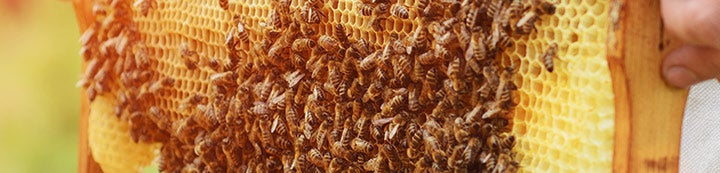### General Information

I have started this showcase to display what my treatments look like and the results I achieve.

#### Ruggggus

·
Currently 18 colonies of mutts (no specific genetics).
Joined
·
47 Posts
Bruce Hovey
2019 OAV treatments
location is upstate NY, hardiness zone 6A

This was my first year attempting to keep track of what is actually happening inside the hive. I had no certain goal that I was attempting to achieve. Was under the impression that 1% was the treatment threshold so I was thinking that once the total count for a treatment was below 250 for a single hive treatment that I was good (I do not think this is an acceptable threshold, it should be much lower).
Hives are all 10 frame Langstroth double deep boxes on a screened bottom board with plasticore pull out trays. The trays are clean of any debris.
All hives are painted a different color.
All 6 hives are strong colonies of 25k bees or more, the white hive is likely 35k.
Note that the yellow hive is queen less and has no brood.
I am dosing with ½ teaspoon of 99.6% pure oxalic acid crystals using an exterior type OAV device.

10/5/19 - treatment
Dead mite counts done on 10/6, 10/7, 10/8, 10/9
Green 16+23+9+6= 54
Gray 18+11+14+7= 50
yellow 65+129+101+72= 367
Lilac 68+133+95+75= 354
Pumpkin 87+53+21+8= 169
White 414+384+72+51= 921

10-Oct Second OAV @ 7:30 am
@ 5:30 pm found a new queen with fresh eggs and young larvae in the top box of the yellow hive.
Mite drop counts performed at 10/10 pm, 10/11 pm, 10/12 2:30, 10/13 4:15

Change
Green 42+89+12+12= 155 up 187.04%
Gray 12+36+15+15= 78 up 56%
Yellow 62+292+109+68= 531 up 44.69%
Lilac 133+427+222+153= 935 up 164.12%
Pumpkin 37+160+29+23= 249 up 47.34%
White 184+606+112+52= 954 up 3.58%
NOTE: the mite drop count was higher than it was for the 1st treatment for every hive.

3rd OAV treatment 10/14 in the am
Mite drop counts performed at 10/15 am and pm, 10/18 am and 10/19 am

Change
Green 15+8+9+2= 34 down 78.06%
Gray 65+67+94+35= 261 up 234.62%
yellow 184+81+94+2= 361 down 32.02%
Lilac 723+330+604+151= 1808 up 93.37%
Pumpkin 180+55+76+23= 334 up 34.14%
White 219+33+141+28= 421 down 55.87%
NOTE: the percentages are all over the place but the yellow hive known to be brood less
till the beginning of the second treatment is showing the improvement that you would expect.

4th OAV treatment 10/19 in the am, Counts at 10/20am, 21pm, 22pm, 23, 24 and 25

Change
Green 24+21+2+1+2+1 =51 up 50%
Gray 68+68+19+9+15+7 =186 down 28.74%
yellow 11+6+5+2+1+2 =27 down 92.52%
Lilac 365+291+116+70+54+24 =920 down 49.12%
Pumpkin 91+45+21+16+18+8 =200 down 40.12%
White 129+121+14+2+5+1 =272 down 35.39%

I have no idea why I stopped the treatments at this time. With my current knowledge I would not stop at this level of mite drop counts.
Though they were all on the downward swing there was a long way to go.

Recap of dead mites counted after 4 OAV treatments
Infestation
Green 54+155+34+51 =294 1.18%
Gray 50+78+261+186 =575 2.30%
yellow 367+531+361+27 =1286 5.14%
Lilac 354+935+1808+920 =4017 16.07%
Pumpkin 169+249+334+200 =951 3.80%
White 921+954+421+272 =2568 7.34% if 35k bees
Apiary total of 9691 6.06%

12/23/2021 checked weight of all 6 hives, cleared out dead bees, treated all with OAV around 3:30. (2 month gap in treatments) counts done on 12/24 1:30 pm12/24 1:30 pm, 25, 26, 27 &28

Green 13+31+34+27+4 =109
Gray 36+51+66+59+8 =220
Yellow 5+7+4+5+1 =22
Lilac 55+143+92+58+18 =366
Pumpkin 34+65+59+22+15 =195
White 1+4+5+4+1 =15

NOTE: Appears that the treatments were sufficient to get the threshold below 1% (except for lilac hive) assuming there is very little brood
at the end of December and the OA has 100% kill rate. Decided to treat again to see what drops.

Treated all 6 hives 12/28 at 9:00am, counts done on 12/29 11 AM, 12/31 noon, 1/1 2PM, 1/3 8 AM.

Change
Green 12+1+0+1 = 14 down 30.28%
Gray 21+5+2+3 = 31 down 94.50%
Yellow 0+0+0+1 = 1 down 95.45%
Lilac 53+17+4+2 = 76 down 79.23%
Pumpkin 13+33+0+2 = 48 down 75.38%
White 1+1+0+1 = 3 down 80.00%

Total of 182 dropped mites, happy with counts, I will not treat again till spring and all colonies are living.
Spring of 2020 revealed that the lilac hive had Chronic Bee Paralysis Virus (CBPV) or something similar, I did not send a sample for testing, which was likely transmitted by the heavy mite load they endured.

·